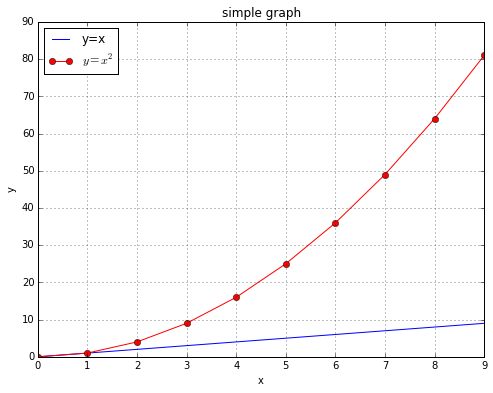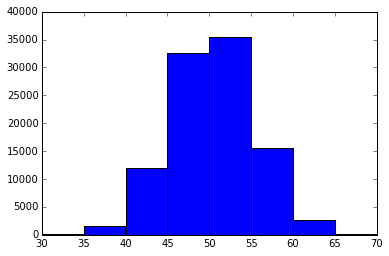In :
print "hello world"

hello world

In :
a='hell'
b='o, w'
c='orld'
print a+b+c

hello, world

In :
d=a+b+c

In :
print d+'!'

hello, world!

In :
d[3:6]

Out:
'lo,'
In :
%pylab inline

Populating the interactive namespace from numpy and matplotlib

In :
arange(10)**2

Out:
array([ 0,  1,  4,  9, 16, 25, 36, 49, 64, 81])
In :
figure(figsize=(8,6))
plot(arange(10))
plot(arange(10)**2,'o-r')
legend(['y=x','$y=x^2$'],loc='upper left')
grid('on')
xlabel('x')
ylabel('y')
title('simple graph');In :
legend?

In :
#see how many of 100 coin flips come up heads
#rand(N) is a list of 100 random numbers between 0 and 1
#sum() in this case counts the number of times the condition is True

sum([r < .5 for r in rand(100)])

Out:
46

stdev for this is sqrt(N*p(1-p))=sqrt(100/4) = 5, so by "68-96-99.7 rule":
roughly 68% of the time between 45 and 55
95.5% of the time between 40 and 60,
and 99.7% of the time between 35 and 65 .
Let's do T=100,000 trials to confirm, then draw a histogram:

In :
T=100000
data=[sum([r < .5 for r in rand(100)]) for t in xrange(T)]
for s in 5,10,15:
print '50 +/-',s,len([d for d in data if 50-s <= d < 50+s])/float(T)

50 +/- 5 0.68044
50 +/- 10 0.95458
50 +/- 15 0.99714

In :
hist(data,bins=range(0,101,5))
xlim(30,70);In [ ]: